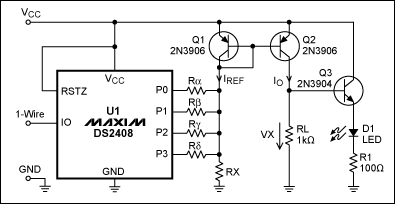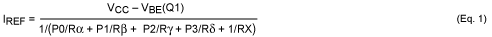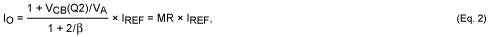A Simple 1-Wire DAC

A Simple 1-Wire DAC

Abstract: ConnecTIng the outputs of a 1-Wire addressable switch (DS2408) to a resistor network allows the 1-Wire interface to control a simple DAC. This idea is illustrated by the presentaTIon of an LED-brightness controller.

A similar version of this arTIcle was published on September 29, 2009 on the Planet Analog website.

Among devices available with a 1-Wire interface, the best known are memories, temperature sensors, battery monitors, and addressable switches. One-Wire ADCs are also available, but no 1-Wire DACs. You can, however, build a simple 1 -Wire DAC using addressable switches.

The circuit (Figure 1) consists of a mulTI-channel 1-Wire addressable switch (DS2408, U1), a current mirror (Q1, Q2), and a resistor network (RÎ±, RÎ², RÎ³, RÎ´, RX). U1's open -drain output transistors (P0-P3) either conduct (logic 0) or present a high impedance (logic 1). If the logic state of an output port is 0, then current flows to ground through the resistor connected to that port.Figure 1. This 4-bit DAC is controlled by a 1-Wire interface. (DS2408 outputs P4 to P7 are not used.)

IREF is calculated as follows:As explained in the ReferenceÂ¹, the current IO is described by equation (2):where VA represents the early voltage of Q2 and Î² is the current gain, assuming Q1 and Q2 have identical characteristics. For VCB values â€‹â€‹from 4.3V to 0.2V and typical values â€‹â€‹for VA (20) and Î² (180), the mirror ratio MR ranges from 1.20 (high VCB, low current) to 1.00 (low VCB, high current).

The DAC's analog output voltage (VX) is
 VX = IO Ã— RL = MR Ã— IREF Ã— RL (Eq. 3)
When none of the output ports are asserting logic 0, IREF is determined by RX:
 IREF0 = (VCC-VBE (Q1)) / RX (Eq. 4)
IREF0 generates the offset voltage VX0:
 VX0 = MR Ã— IREF0 Ã— RL (Eq. 5)
Inserting (4) in (5) and solving for RX yields (6), which lets you calculate the RX value corresponding to a desired offset voltage:
 RX = RL Ã— MR Ã— (VCC-VBE (Q1)) / VX0 (Eq. 6)
With P0 assigned to the DAC's least-significant bit (LSB), the resistor RÎ± determines Î”VX, which is the voltage increment between steps. Substituting Î”VX for VX0 in equation (6) yields (7), which lets you calculate RÎ±:
 RÎ± = RL Ã— MR Ã— (VCC-VBE (Q1)) / Î”VX (Eq. 7)
Because P1 has twice the weight of P0 in a binary system, RÎ² must conduct twice as much current. Consequently, RÎ² = RÎ± / 2. For the same reasons, RÎ³ = RÎ± / 4 and RÎ´ = RÎ± / 8. For these conditions the maximum value of VX is
 VXMAX = VX0 + 15 Ã— (VCC-VBE (Q1)) / RÎ± Ã— RL Ã— MR (Eq. 8)

Table 1. Input Values â€‹â€‹for the Full-Range DAC
 Parameter Explanation VCC = 5V Maximum voltage for the DS2408 VX0 = 0.05V Design objective Î”VX = 0.25V Design objective VBE (Q1) = 0.7V Simplification MR = 1.1 Center of the 1.00-to-1.20 MR range RL = 1kÎ© To ensure operation with high current gain

Example 2: LED-Brightness ControlIn Figure 1, the analog output voltage VX controls the light intensity of an LED. Q3 functions as an emitter follower that minimizes the load on VX, and R1 limits the LED current. Assume that the LED voltage drop is 1.9V when ON, and that VBE of Q3 is 0.7V. To keep the LED OFF, VX must be less than 2.6V, ie, VX0 = 2.5V. To avoid Q2's VCE saturation range, the voltage swing at VX must remain below 2V. This condition demands that Î”VX â‰¤ 2V / 15, ie, Î”VX = 0.125V. Otherwise, the input values â€‹â€‹of Table 1 apply.